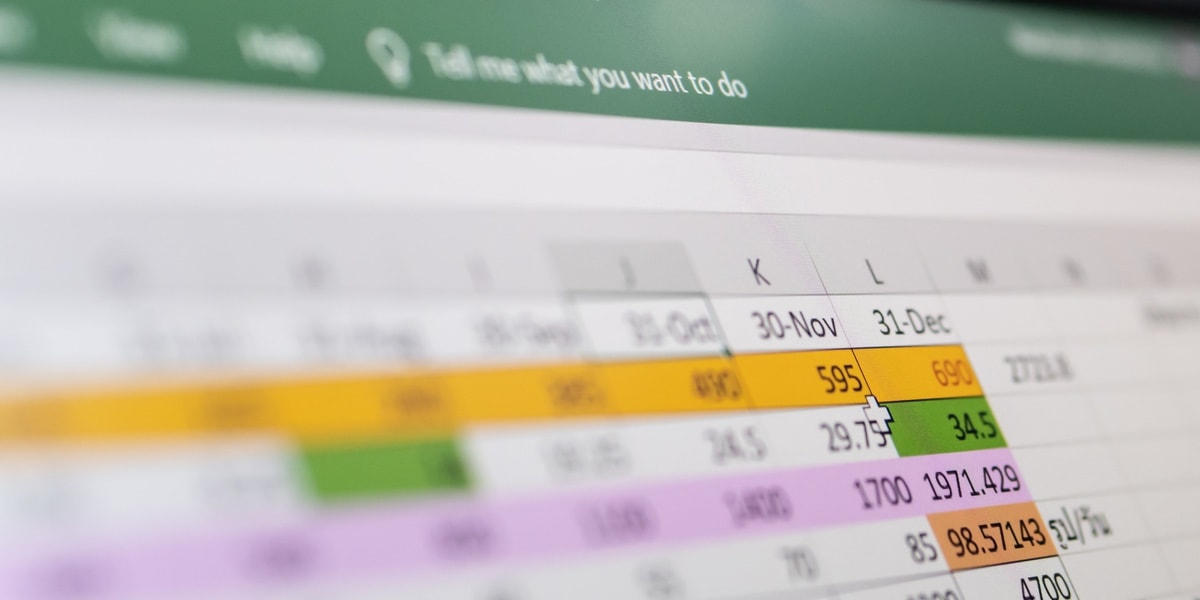Microsoft Excel, that tricky beast we’ve all used at some point in our lives, is an essential tool in data analytics. This spreadsheet program is one of the first things aspiring data analysts must get to grips with. In this article, we’re giving you a taste of how Excel is used by data analysts.

To use Excel properly, a good understanding of the program’s formulas is required. These formulas operate on values within a cell or cells, producing specific information such as the total sum or lowest value of a selection of cells. We’re launching an Intro to Data Analytics Course, which will focus on a series of Excel-based analytical tasks. The course is comprised of 10 exercises, and students will learn the basics of Excel while working on a data set related to the video game industry.

To get you in the mood for some number crunching, we’re covering 10 Excel formulas that’ll make your life a lot easier if you learn them.  By the end of this article, you’ll have an understanding of the basic functions of Excel, and an appreciation for its capabilities. Let’s dive straight in.

2. VLOOKUP

3. LEN

4. SUMIFS

8. TRIM

9. COUNTIFS

10. LEFT/RIGHT

### 1. CONCATENATE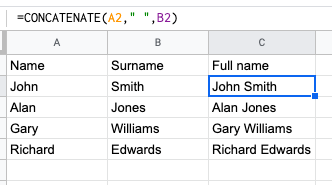Let’s start with a straightforward one. Concatenate allows you to combine the values of several cells into one. It’s one of the most powerful formulas for conducting data analysis, and is commonly used to combine text, dates and numbers. Combining the components of a URL, or the lines of an address, are two typical examples of what the formula is used for.

### 2. VLOOKUP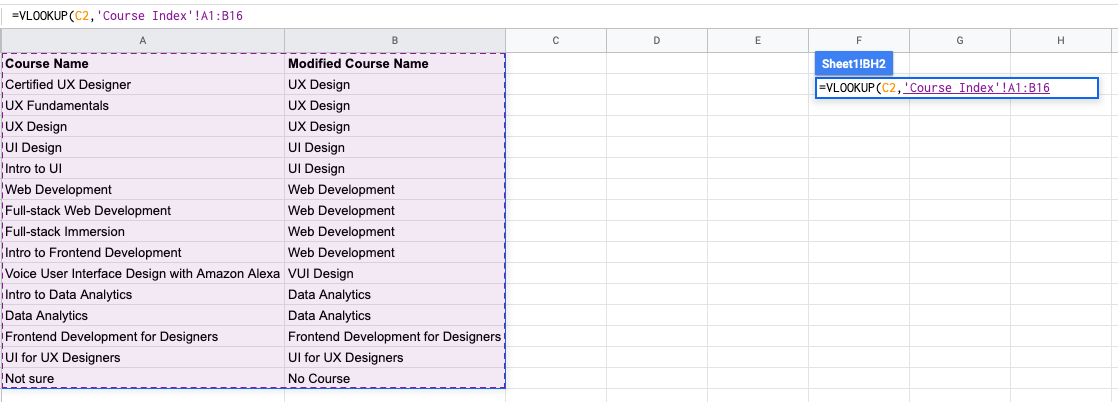You’ve no doubt come across =VLOOKUP, which will be familiar to anyone who’s used Excel. The formula allows you to look up data that is arranged in vertical columns. For example, if you have a list of months of the year with the profit made in each month, =VLOOKUP can present the data from the month of your choice.

### 3. LENLEN is used in data analysis to display the number of characters in any given cell. It’s often used when creating texts with character limits, or when trying to identify the difference between product numbers. In the above example, Len is used to count the number of characters in the names.

### 4. SUMIF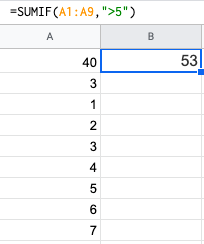The =SUMIF function is an essential formula in the world of data analytics. The formula adds up the values in cells which meet a selected number. In the above example, the formula is adding up the numbers in cells that are higher than the number 5.

### 5. DAYS/NETWORKDAYS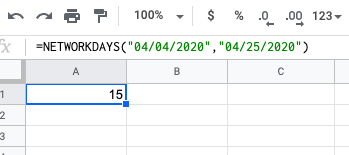This one is fairly self explanatory. =DAYS determines the number of days between two calendar dates, and is commonly used to determine product life cycles or contract periods. =NETWORKDAYS is its more useful counterpart, and is used to calculate the number of working days between two dates. You could say this formula lives for the weekend.

### 6. SUBSTITUTE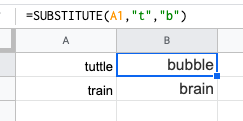This is a useful trick that is ideal for updating cells in bulks. It’s another one that does exactly what it says, and is extremely useful for updating URLs, removing unintentional spaces or spelling errors. In the example above, the formula is replacing the letter ‘t’ with ‘b’.

### 7. MINIFS/MAXIFSThis handy formula identifies which value is the lowest and which is the highest. But it does more than just that, it also sorts values in relation to a particular criteria too. For example, you can use it to sort the oldest and youngest ages from a sample of men and women, displaying the values by gender. In the above example, the MINIFS formula is returning 88, because that’s the lowest number that meets the criteria.

### 8. TRIMUsing =TRIM allows you to remove unwanted spaces or characters from text. In order for analysts to work with data, often you must clean up the data before use. =TRIM is one of the many useful formulas that cleans up data before analysis. The example above shows how an unwanted space was removed at the end of a name.

### 9. COUNTIFSTHE COUNTIFS formula counts the numbers how many times a value appears based on one criteria. In the example above, ‘Red’ and ‘England’ appear twice together, and that number is returned in the COUNTIFS cell.

### 10. LEFT/RIGHT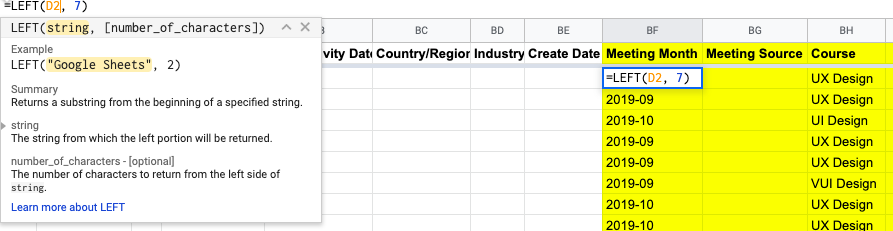The =LEFT and =RIGHT formulas are simple ways of getting data out of static cells. =LEFT returns the desired number of characters from the beginning of the cell, while =RIGHT does the opposite. Need to extract the area codes from a list of phone numbers? This is the formula for you.

### Final thoughts

There are many analysis tools available within specific programming languages, but Excel is a popular choice when analyzing relatively small amounts of data. The 10 formulas we’ve covered represent the tip of the iceberg in terms of what you can do with Excel, but they give you a taste of the kind of powers you can yield as a data analyst.

If this has whetted your appetite for more, you’ll find a hands-on introduction to data analytics in this free five-day short course. And, if you’d like to learn more about forging a career as a data analyst or data scientist, check out the following:

### What You Should Do Now

1. Get a hands-on introduction to data analytics with a free, 5-day data analytics short course.
2. Take a deeper dive into the world of data analytics with our Intro to Data Analytics Course.
3. Talk to a program advisor to discuss career change and find out if data analytics is right for you.
4. Learn about our graduates, see their portfolio projects, and find out where they’re at now.